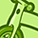# QlikView App Dev

Discussion Board for collaboration related to QlikView App Development.

Announcements
Join “Do More With Qlik” with Mike Tarallo to get a first-hand look at the New Authoring Experience, June 29th: REGISTER HERE
cancel
Showing results for
Did you mean:Creator

## Unable to use alternate state or filter the dimension while using set analysis for previous calendar / fiscal year

Sample data set :

DistributorFY 2018-2019
Value
FY 2017-2018
Value
FY 2018-2019
Value Contribution
FY 2017-2018
Value Contribution
a6,666.0567.09.1%3.1%
ABC4,563.05,432.06.2%29.8%
c1,111.0789.01.5%4.3%
d2,222.0987.03.0%5.4%
f3,333.0563.04.5%3.1%
e55,555.09,871.075.6%54.2%

variables :

v_FYear = if(getselectedcount(FiscalYear)=1,1,0)

v_Year=if(getselectedcount(Year)=1,1,0)

-----------------------------------------------------------------------------------------------------------------------------------------------------------------

Hello All,

I'm trying to filter the dimension (Distributor) by using Alternate state or by using the directly using the "Distributor.name " in the if condition

1.METHOD 1 : using the directly the "Distributor.name " in the if condition

 =if(Distributor.Name='ABC' and v_Year=1,Sum({\$ [Value] )/100000,'')

Below is the screenshot of the output I get :---------------------------------------------------------------------------------------------------------------------------------------------------------------------

2. METHOD 2 : By using the Alternate State

Alternate_state1 is the list box for the Distributor names

Here is syntax that I used  for alternate state method to get the previous year value in the expression

=if(v_Year=1,Sum({\$<Year={\$(=max(Year)-1)}>}{[Alternate_State1]*\$}[Value])/100000,if(v_FYear=1,sum({\$<[FiscalYear]={"\$(=Only((SubField([FiscalYear],'-',1) - 1) & '-' & (SubField([FiscalYear],'-',2) - 1) ))"}>} {[Alternate_State1]*\$}[Value] )/100000,''))

The above atlernate state method is used to output the previous calendar / fiscal year , but my output shows the Current year instead of Previous year .

------------------------------------------------------

Here is syntax that I used  for alternate state method to get the previous year share of business in the expression

=if(v_Year=1 ,

(sum({\$<Year={\$(=max(Year)-1)}>}{[Alternate_State1]*\$}[Value])/sum({\$<Year={\$(=max(Year)-1)}>}TOTAL[Value])),

if(v_FYear=1,

sum({\$<[FiscalYear]={"\$(=Only((SubField([FiscalYear],'-',1) - 1) & '-' & (SubField([FiscalYear],'-',2) - 1) ))"}>} {[Alternate_State1]*\$}[Value] )

/sum({\$<[FiscalYear]={"\$(=Only((SubField([FiscalYear],'-',1) - 1) & '-' & (SubField([FiscalYear],'-',2) - 1) ))"}>} Total[Value] )))

- the above expression calculates current years value / previous year total value which is wrong as I need previous year divided by previous year total

-

++++++++++++++++++++++++++++++++++++++++++++++++++++++++++++++++++++++++++++++++++++++++++++++++++++